Next: Combining Probabilities Up: Probability Theory Previous: Introduction

# What is Probability?

What is the scientific definition of probability? Well, let us consider an observation made on a general system,. This can result in any one of a number of different possible outcomes. Suppose that we wish to find the probability of some general outcome,. In order to ascribe a probability, we have to consider the system as a member of a large set,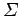, of similar systems. Mathematicians have a fancy name for a large group of similar systems. They call such a group an ensemble, which is just the French for group.'' So, let us consider an ensemble,, of similar systems,. The probability of the outcomeis defined as the ratio of the number of systems in the ensemble which exhibit this outcome to the total number of systems, in the limit that the latter number tends to infinity. We can write this symbolically as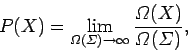(1)

where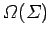is the total number of systems in the ensemble, and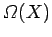the number of systems exhibiting the outcome. We can see that the probability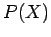must be a number between 0 and 1. The probability is zero if no systems exhibit the outcome, even when the number of systems goes to infinity. This is just another way of saying that there is no chance of the outcome. The probability is unity if all systems exhibit the outcomein the limit as the number of systems goes to infinity. This is another way of saying that the outcomeis bound to occur.Next: Combining Probabilities Up: Probability Theory Previous: Introduction
Richard Fitzpatrick 2010-07-20